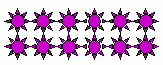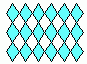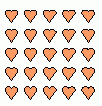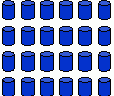Email us to get an instant 20% discount on highly effective K-12 Math & English kwizNET Programs!

#### Online Quiz (WorksheetABCD)

Questions Per Quiz = 2 4 6 8 10

### MEAP Preparation - Grade 7 Mathematics1.91 Percentages For Numbers Using Pictures

 Example: 25% means 25 out of 100, or 1 out of 4. Therefore, if you have a picture showing 16 items, mark 1 out of every 4 items to get 25% of 16. Mathematically, 25% of 16 is (25/100)x16 = 4 Directions: Use the pictures to find the required percentages. Also write at least ten examples of your own.
 Q 1: What is 30% of 40?41012 Q 2: What is 50% of 16?1284 Q 3: What is 25% of 12?346 Q 4: What is 50% of 18?9510 Q 5: What is 20% of 25?51015 Q 6: What is 75% of 24?12618 Question 7: This question is available to subscribers only! Question 8: This question is available to subscribers only!